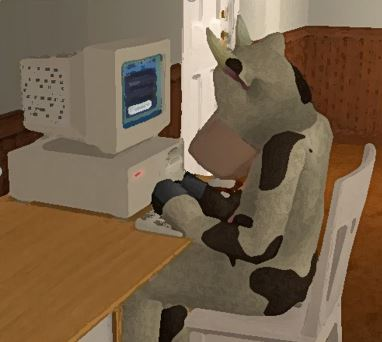## Problem 4: Server HackingThe Center for Exploration of Monkey Cryptography (CEMC) is an organization dedicated to wartime cryptography research amongst the primate community. As the monkeys are once again at war with the cows, this institution has become indispensable to their success in combat. After all, taking over Scarberia in the 21st century will require some of the most sophisticated code-breaking techniques in existence to infiltrate the enemies' communications. As it turns out, the cows possess a slight technological edge over the monkeys, due to their experimentation with Cow-Bots in 2002. They were therefore able to reverse engineer the structure of the CEMC's servers!

The cows have learned that the server is a network consisting of N (1 ≤ N ≤ 100,000) computers arranged in a line topology. Specifically, the computers are numbered from 1 to N, and are connected in a line in ascending order. For example, computer 1 is connected only to computer 2, computer 2 is connected only to computers 1 and 3, and computer N is connected only to computer N − 1. The network is encrypted using a simple public key cryptosystem. Each computer i has a distinct public key, an integer Ai (1 ≤ Ai ≤ 109). The private key to access computer i is a sequence of integers based on the prime factorization of Ai. Consider Ai = 1200 with the prime factorization of 24 × 31 × 52 when the prime factors are listed in ascending order. Its private key is then the sequence [2, 4, 3, 1, 5, 2].

Typical brute force attacks rely on trying every possibility to gain access. In this case, a private key is easier to guess if it comes "earlier" in an exhaustive list of possible guesses. So for two different computers i and j, we will call the public key of i weaker than the public key of j if and only if the private key sequence of i is lexicographically smaller than the private key sequence of j. A sequence X is considered to be lexicographically smaller than another sequence Y if Xi < Yi for the smallest index i such that Xi differs from Yi. If every value in X is equal to the values at corresponding indices in Y and X is a shorter sequence (i.e. if X is a prefix of Y), then X is also considered lexicographically smaller than Y. For example, [2, 2, 7, 1] is lexicographically smaller than [2, 3] and [2, 3] is lexicographically smaller than [2, 3, 5, 7].

Having discovered the weak cryptographic scheme of the CEMC's servers, the cows now wish to assert their dominance and generate chaos amongst the monkeys by performing a distributed denial-of-service (DDOS) attack on the network to take it down. However, they will need a weakpoint from which to initiate the attack. In particular, a weakpoint is a computer whose public key is weaker than that of every other computer that it is directly connected to. Please help the cows write a program to find a weakpoint and crash the CEMC's servers. If there are multiple such weakpoints, then any one of them will suffice.

In test cases worth 5/25 of the points: N ≤ 1000.

### Input Format

The first line of input consists of a single integer N.
N lines follow, with the i-th of these lines containing Ai (for i = 1..N).

### Output Format

Output a single integer between 1 and N, your chosen weakpoint.

```4
840350
341796875
1584
735166567
```

```1
```

### Explanation

Computer 1's public key has the prime factorization 840350 = 21 × 52 × 75, so its private key is [2, 1, 5, 2, 7, 5].
Computer 2's public key has the prime factorization 341796875 = 511 × 71, so its private key is [5, 11, 7, 1].
Computer 3's public key has the prime factorization 1584 = 24 × 32 × 111, so its private key is [2, 4, 3, 2, 11, 1].
Computer 4's public key has the prime factorization 735166567 = 267831 × 274491, so its private key is [26783, 1, 27449, 1].
Therefore, both computers 1 and 3 are valid weakpoints that will be accepted.

Point Value: 20 (partial)
Time Limit: 0.20s
Memory Limit: 32M
Author: Alex

Languages Allowed:
C++03, PAS, C, HASK, ASM, RUBY, PYTH2, JAVA, PHP, SCM, CAML, PERL, C#, C++11, PYTH3

• (0/0)
In an attempt to filter out suboptimal algorithms, the time limit for this problem on the main judge has been lowered to 0.2s.

• (0/0)
Is it still possible to do the problem in Python?

• (0/0)
Is tle(wall clock) used here to enforce the strict time limit, or is it an actual problem?

• (0/0)
Elapsed time limit is 5 times CPU time limit (which is currently 0.2 s for this problem). It can't be set separately. It's nothing more than a mechanism to prevent submissions from taking forever.